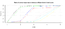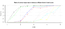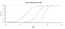# R graphs

## R graphs

Ετικέτες: RMobility_8445_samples_score_probability.png (png) [Image Details]
item characteristic curves from all the dataset (8445 samples) based on ltm model.
55 kBMobility_8445_samples_item_characteristic_curves.png (png) [Image Details]
item characteristic curves from all the Mobility dataset (8445 samples) without fit to a model. x axis: score (summary) y axis: probability
49 kBMobility_600_samples_score_probability.png (png) [Image Details]
item characteristic curves from a random sample of Mobility dataset (600 samples) without fit to a model. x axis: score (summary) y axis: probability
56 kBMobility_600_samples_item_characteristic_curves.png (png) [Image Details]
item characteristic curves from a random sample of Mobility dataset (600 samples) based on ltm model.
47 kB